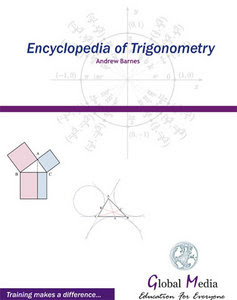Encyclopedia of Trigonometry
Publisher: Global Media | ISBN: 9788189940010 | edition 2008 | File type: PDF | 140 pages | 15,3 mb

“Introduction” In Simple Terms” Radian and Degree Measure” Trigonometric Angular Functions” Right Angle Trigonometry” Properties of the cosine and sine

functions” Taylor series approximations for the trig. functions” Inverse Trigonometric Functions” Applications and Models” Verifying Trigonometric Identities” Solving Trigonometric Equations” Sum and Difference Formulas” Additional Topics in Trigonometry” Solving Triangles” Vectors in the Plane” Trigonometric Identities” History of trigonometry” Common formulae” Exact trigonometric constants” Pythagorean theorem